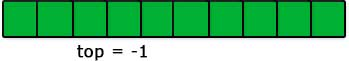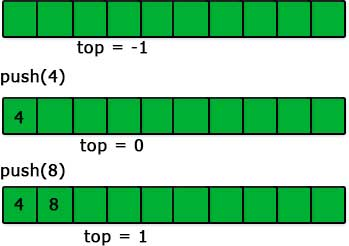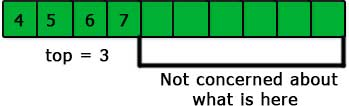# Making a stack using an array in C

May 26, 2017 41819

### Previous:

The previous article was all about introducing you to the concepts of a stack. In this article, we will code up a stack and all its functions using an array.

As we know that we can’t change the size of an array, so we will make an array of fixed length first (this will be the maximum length of our stack) and then implement stack on it.

So, let’s do this.

#include <stdio.h>
#define SIZE 10

int stack[SIZE];


Here, we have created an array name ‘SIZE’ of length ‘SIZE’. This ‘SIZE’ is the maximum length of our stack.

The next thing to do to create a stack is the creation of the ‘top’ pointer. This pointer will point to the top element of the stack. In the implementation using an array, this ‘top’ pointer will be a non-negative integer which will always be equal to the index of the topmost element of the stack and if the stack is not initialized then we can make it some negative integer (we will make -1 in this article).

#include <stdio.h>
#define SIZE 10

int stack[SIZE];
int top = -1;


You can see that we have made the ‘top’ equal to -1 because the stack is not yet initialized.The next and the most important operations on a stack are push and pop. So, let’s create them.

### push

The steps for push operation are:

1. Check if the ‘top’ is negative or not.
2. If the ‘top’ is negative then make it 0 and put the ‘value’ in the element having 0 index in the array ‘stack’.
3. If it is not then put the value in the element having index ‘top+1’ in the array ‘stack’ and increase the top by 1.These are very simple and logical steps. Let’s code these steps and implement the push operation.

void push(int value)
{
if(top<SIZE-1)
{
if (top < 0)
{
stack = value;
top = 0;
}
else
{
stack[top+1] = value;
top++;
}
}
else
{
printf("Stackoverflow!!!!\n");
}
}


if(top<SIZE-1) – We are just making sure that our stack is not full and we can insert more items.

if (top < 0)/*step 1*/ {  stack = value;/*step 2*/  top = 0;/*step 2*/ } else {  stack[top+1] = value;/*step 3*/  top++;/*step 3*/ }
We have just follow the steps mentioned above with these lines of code.

One point to note here is that we only care about the element present till the index 'top' in the stack. We are not concerned with what is present after the index 'top' because they are not the part of our stack.### pop

In pop operation, we return the topmost element and then decrease the value of the ‘top’ by 1. That’s it. Let’s do this.

int pop()
{
if(top >= 0)
{
int n = stack[top];
top--;
return n;
}
}


This is a very simple code. Firstly, we are just making sure that the stack is not empty and then decreasing the ‘top’ by 1 and returning the previous topmost element.

Thus, the overall code for the implementation of stack using array is:

#include <stdio.h>
#define SIZE 10

int stack[SIZE];
int top = -1;

void push(int value)
{
if(top<SIZE-1)
{
if (top < 0)
{
stack = value;
top = 0;
}
else
{
stack[top+1] = value;
top++;
}
}
else
{
printf("Stackoverflow!!!!\n");
}
}

int pop()
{
if(top >= 0)
{
int n = stack[top];
top--;
return n;
}
}

int Top()
{
return stack[top];
}

int isempty()
{
}

void display()
{
int i;
for(i=0;i<=top;i++)
{
printf("%d\n",stack[i]);
}
}

int main()
{
push(4);
push(8);
display();
pop();
display();
return 0;
}


## Comparing stack using linked list and stack using array

• Array implementation is easy.
• Array implementation has limited capacity because of using a fixed size array.
• Array implementation requires less space.

Liked the post?
Developer and founder of CodesDope.
0 COMMENT